• # 9 Search Results 'Yunus Cengel'

• 0

Filter(s)

### All 9 results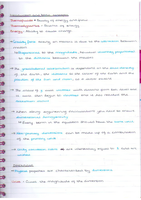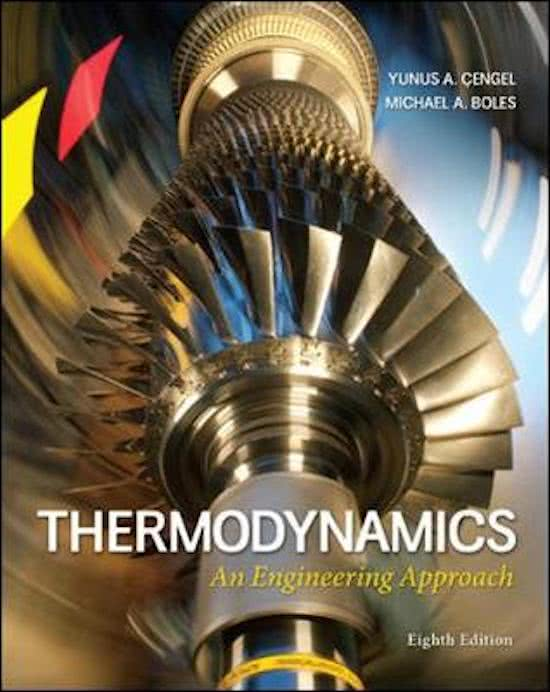#### Intro to thermodynamics and pure substances

(0)
\$27.50
0x  sold

These notes are a combination of lecture notes and notes from the textbook Thermodynamics: An engineering approach. There are 81 pages which include definitions, explanations, diagrams, keywords are highlighted and there are a few example calculations provided as well. The topics in these notes are the basics, pressure, fluids, pure substances and the first law.

•  Book
• Class notes
•  • 1 pages •
• by lchand1 •
Quick View
i x
•
•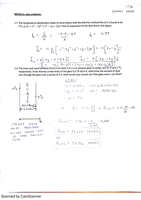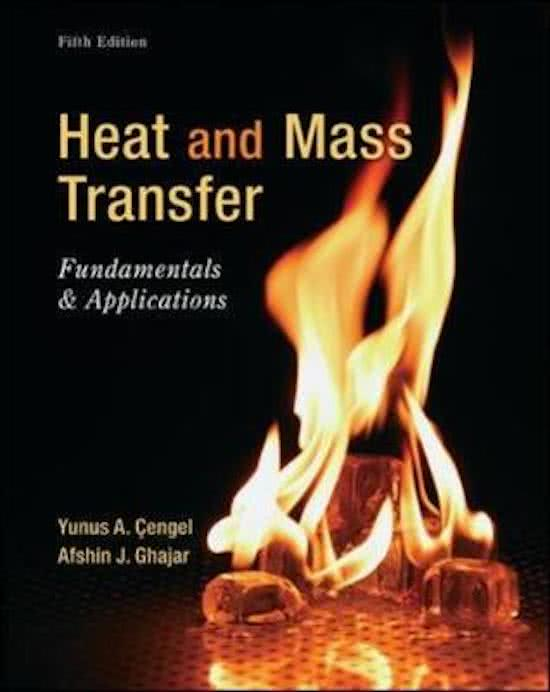#### Heat Transfer Day 3

(0)
\$5.49
0x  sold

These lecture notes include two class problems on conduction. The first problem shows one way to find the heat flux of an object with a given temperature distribution. The second problem shows how doubling a window's thickness can impact its rate of heat loss. The class notes give the heat conduction (Fourier's) equation in one dimension and the definition of the gradient operator (upside-down delta).

•  Book & Bundle
• Class notes
•  • 3 pages •
• by jerry404 •
Quick View
i x
•
•
•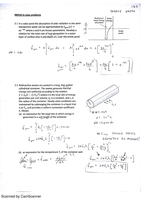#### Heat Transfer Day 5

(0)
\$5.49
0x  sold

There are two in-class problems; 1. Given a pond and its rate of heat generation per unit mass, the rate of heat generation for the whole pond is found (in variable form). 2. For a cylindrical container holding radioactive waste, heat is generated at a given rate per unit mass (indicated by e). Expressions for the heat generation rate of the whole container and the temperature at its outer surface are found. The in-class notes review the equations for convection and radiation. Also, a couple sou...

•  Book & Bundle
• Class notes
•  • 3 pages •
• by jerry404 •
Quick View
i x
•
•
•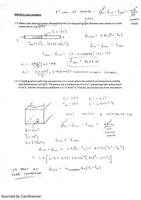#### Heat Transfer Day 4

(0)
\$5.49
0x  sold

Includes two class problems; first shows the heat generation in an electrical wire and second shows the heat lost from a person to a room due to convection and radiation. The first law of thermo is applied to both problems and will continue to be a valuable approach. The class notes touch more on convection and radiation with their equations and the definitions of the variables in them.

•  Book & Bundle
• Class notes
•  • 3 pages •
• by jerry404 •
Quick View
i x
•
•
•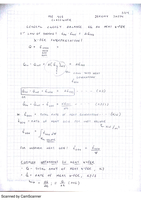#### Heat Transfer Day 2

(0)
\$5.49
0x  sold

Shows the first law of thermodynamics, total energy generation rate for a volume, heat flux and the three ways in which heat is transferred. First law (a.k.a. energy balance) is expressed two ways; first, as a change from an initial time to a final time and second, on a per unit time basis (derivative form). Mentions the three ways heat is naturally transferred between materials.